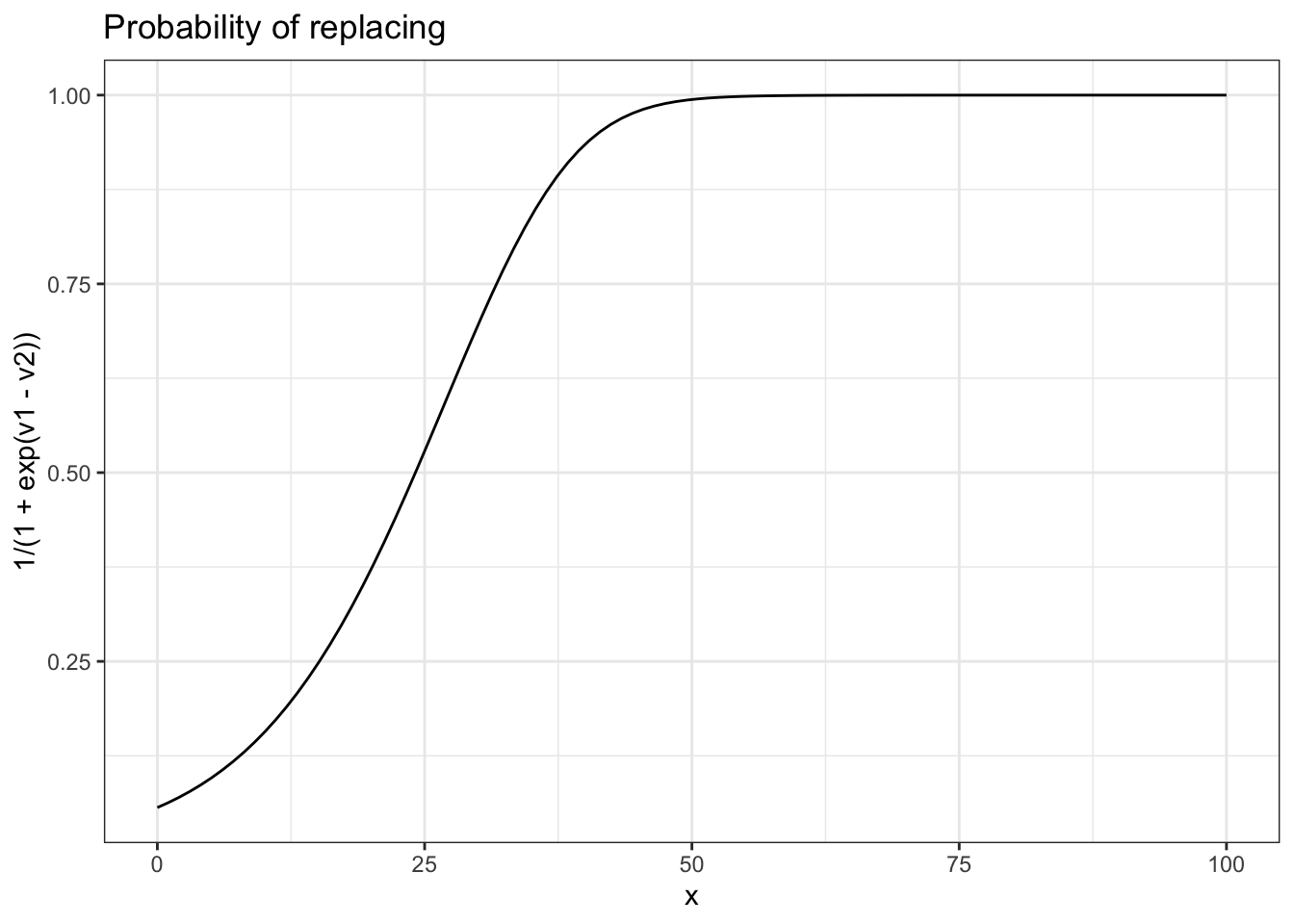1. Consider a simple saving problem (cake eating)
2. Explain Bellman problem without uncertainty
3. Explain Bellman problem with uncertainty
4. Solve simple consumption saving problem
5. Simulate transitory and permanent policies
6. Plot a fit using some simple distance, an indirect inference estimator

We consider here discrete choices over a set of alernatives. The utility of the agent is modeled as

# Cake eating

Imagine you a given a cake os size $$S$$, and you need to decide how much to eat everyday. How would you formulate the problem?

$\max_{c_t} \sum_{t=0}^\infty \beta^t u( c_t) \quad s.t. \quad \sum_{t=0}^\infty c_t \leq S$ One can write the Lagrangian of this problem and find:

$L = \sum_{t=0}^\infty \beta^t u( c_t) - \lambda \Big( \sum_{t=0}^\infty c_t - S \Big)$

This gives the following FOCs:

$\beta^t u'(c_t) = \lambda$

Consider a CRRA utility function $$u(c)=\frac{c^{1-\gamma}}{1-\gamma}$$ which gives $$u'(c)=c^{-\gamma}$$ and hence $\sum_{t=0}^\infty c_t = \sum_{t=0}^\infty \Big( \frac{\lambda}{\beta^t} \Big)^{-\frac{1}{\gamma} } = \frac{\lambda^{-\frac{1}{\gamma}}}{1-\beta^{1/\gamma-1}} =S$ hence we get that the consumption will be

$c_t = \Big( \frac{\lambda}{\beta^t} \Big)^{-\frac{1}{\gamma} } = (1-\beta^{1/\gamma-1})\beta^{t/\gamma}S$

As an exercise, one can plot this for different values of $$\beta$$ and $$\gamma$$.

library(data.table)
library(ggplot2)
set.seed(4325342)

## A recursive formulation

Let’s introduce $$V(S)$$ as the present value, the life time value of having a cake of size $$S$$ today and consuming optimally in the future.

$V(S) = \max_{c_t} \sum_{t=0}^\infty \beta^t u( c_t) \quad s.t. \quad \sum_{t=0}^\infty c_t \leq S$ A simple derivation establishes that:

$V(S) = \max_{c} u(c) + \beta V(S-c)$

# The bus engine problem

Imagine that we want to model the decision to replace the engine on in a fleet of buses. This is the premise of a seminal paper by John Rust Optimal Replacement of GMC Bus Engines: An Empirical Model of Harold Zurcher. Here is a simple version of it:

• the state is $$x_t$$, the mileage of the engine
• every period, the bus delivers profit as a function of $$x_t$$, call it $$p(x_t)$$
• the bus owner Mr Zurcher, can decide to replace the engine at cost $$C$$ in which case $$x_t$$ goes back to $$0$$
• the bus dies with probability a function of $$x_t$$ in which case the owner gets $$0$$
• the owner discounts at rate $$\beta$$
• every period, the bus accumulates a $$u$$ miles

Using our Bellman tool we can write the optimal decision for this problem recursively:

$V(x) = \max \{ p(x) + \lambda(x)\beta V(x+u) , -C + \beta V(0) \}$ We can see that this will have a threshold property. In practice the decision won’t be as clear as a simple threshold. We want to allow for some randomness. We then add a logit preference shock $$\xi$$ to the different between the two options. We then remember that we can transform the max into a log-sum-exp.

$V(x) = \log\Big( \exp \Big[p(x) + \lambda(x)\beta V(x+u)\Big] + \exp \Big[ -C + \beta V(0) \Big] \Big) + \gamma$

There is not hope to solve this problem in closed form. We tackle it numerically.

n      = 100 # number of grid point for V
V      = rep(0,n)
x      = seq(0,100,l=n)
lambda = 1/(1+exp(0.1*(x-50))) # break probability
beta   = 0.9
C      = 2
P      = exp(-0.01*x) # profit function
In = c(2:n,n)         # increasing x by 1

# Solving the Bellman equation using a fix point approach
rr = data.frame()
for (i in 1:300) {
rr = rbind(rr,data.frame(x=x,V=V,rep=i,v1=P + lambda*beta*V[In],v2=-C + beta*V ))
V2 = log( exp( P + lambda*beta*V[In]) +  exp(-C + beta*V )  ) + 0.56
dist = mean((V2-V)^2)
V=V2
}

# plotting different iterations
rr = data.table(rr)
ggplot(rr[rep<100],aes(x=x,y=V,group=rep,color=factor(rep))) +
geom_line() + ggtitle("Belman iteration for V") + theme_bw() + theme(legend.position="none")# plotting each options
ggplot(rr[rep %in% c(5,10,60)],aes(x=x,y=v1,group=rep,color=factor(rep))) +
geom_line(linetype=2) +
geom_line(aes(y=V)) +
geom_hline(aes(yintercept =v2,color=factor(rep)) ,linetype=2) +
theme_bw() +
ggtitle("plotting value of replacing versus not")# we can look at the probability to replace
rr2 = data.frame(x=x, V=V, rep=i, v1=P + lambda*beta*V[In], v2=-C + beta*V )
ggplot(rr2,aes(x=x,y=1/(1+exp(v1-v2)))) + geom_line() +
theme_bw() +
ggtitle("Probability of replacing")# final value
ggplot(rr2,aes(x=x,y=V)) + geom_line() +
theme_bw() +
ggtitle("Value function")# simulating data

We simulate a fleet of 100 buses, each over 100 periods.

PR1 = 1/(1+exp( P + lambda*beta*V[In] - (-C + beta*V)))

rr = data.frame()
for (i in 1:100) {
rrr = data.frame()
x=1
l=1
R=0
for (t in 1:100) {

if (l==0) {
rrr = rbind(rrr,data.frame(i=i,t=t,x=0,l=0,profit=0,R=0))
next
}

# draw the maintenance choice
if (PR1[x]>runif(1)) {
R=1
profit = -C

} else {
R=0
profit = P[x]

if (lambda[x]<runif(1)) {
l=0
}
}
rrr = rbind(rrr,data.frame(i=i,t=t,x=x,l=1,profit=profit,R=R))
if (R==1) {
x=1
} else {
x= x+1
}
}
rr = rbind(rr,rrr)
}

data = data.table(rr)
data[,sum(l==1),i][,mean(V1)]
##  68.72
ggplot(data[i==5],aes(x=t,y=profit)) + geom_line() + theme_bw()ggplot(data[i==1],aes(x=t,y=x)) + geom_line() + theme_bw()We take a look at the raw data

data
##          i   t x l    profit R
##     1:   1   1 1 1 1.0000000 0
##     2:   1   2 2 1 0.9899498 0
##     3:   1   3 3 1 0.9800007 0
##     4:   1   4 4 1 0.9701515 0
##     5:   1   5 5 1 0.9604013 0
##    ---
##  9996: 100  96 0 0 0.0000000 0
##  9997: 100  97 0 0 0.0000000 0
##  9998: 100  98 0 0 0.0000000 0
##  9999: 100  99 0 0 0.0000000 0
## 10000: 100 100 0 0 0.0000000 0

Eventually all buses die, we can plot the survival probability:

plot( data[, mean(R), by=t]\$V1)# Writing a likelihood

We can write the likelihood of the decision and miles and sum across individuals

lik <- function(theta) {
n      = 100
V      = rep(0,n)
x      = seq(0,100,l=n)
lambda = 1/(1+exp(0.1*(x-50)))
beta   = 0.9
C      = theta
P      = exp(-0.01*x)
In = c(2:n,n)

rr = data.frame()
for (i in 1:100) {
rr = rbind(rr,data.frame(x=x,V=V,rep=i,v1=P + lambda*beta*V[In],v2=-C + beta*V ))
V2 = log( exp( P + lambda*beta*V[In]) +  exp(-C + beta*V )  ) + 0.56
dist = mean((V2-V)^2)
V=V2
}
Pr  =1/(1+exp( P + lambda*beta*V[In] - (-C + beta*V)))
data[l==1,sum(log(Pr[x])*(R==1) + log(1-Pr[x])*(R==0)) ]
}

V = sapply(seq(1,3,l=30),lik)
plot(seq(1,3,l=30),V)